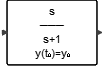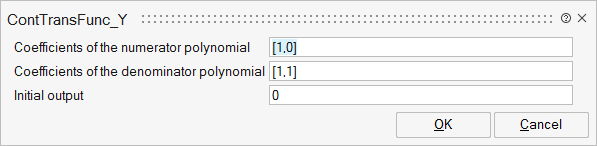# ContTransFunc_Y

This block implements a SIMO (continuous-time, single-input, multi-output) linear system that is defined by the linear system's transfer function. The transfer function, specified by the coefficients of the function's numerator and denominator, must be proper, meaning that the degree of the numerator must not exceed that of the denominator. The internal state of the block is initialized so that the initial output corresponds to the specified value.## Description

This block implements a SIMO (continuous-time, single-input, multi-output) linear system that is defined by the linear system's transfer function. The transfer function, specified by the coefficients of the function's numerator and denominator, must be proper, meaning that the degree of the numerator must not exceed that of the denominator. The internal state of the block is initialized so that the initial output corresponds to the specified value.

## ParametersNameLabelDescriptionData TypeValid Values

num

Coefficients of the numerator polynomial

An mxn matrix where m is the size of the output, and n is the order of the numerator polynomial.

Matrix

den

Coefficients of the denominator polynomial

Defines the denominator of the transfer function.

Vector

y0

Initial output

Vector

## Ports

NameTypeDescriptionIO TypeNumber

Port 1

explicit

output

1

Port 2

explicit

input

1

NameValueDescription

always active

yes

direct-feedthrough

no

no, unless the order of numerator is equal to the order of denominator.

zero-crossing

no

mode

no

continuous-time state

yes

discrete-time state

no